Reflection (mirror) symmetry of cube Materials: 3 mirrors, square fragments, dry erase markers (4 colours)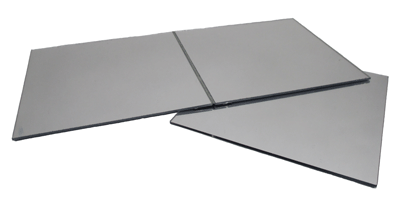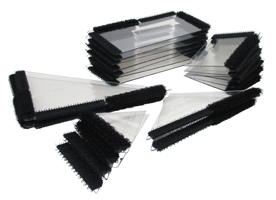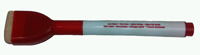First mirror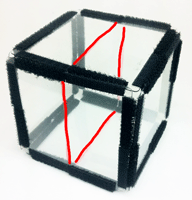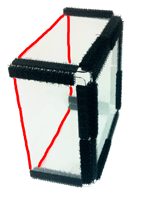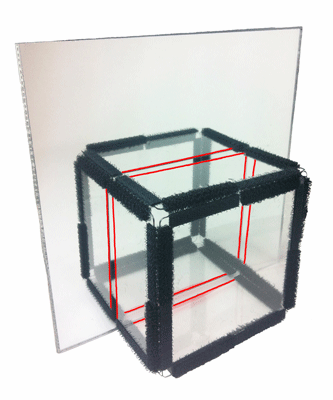The mirror shows a plane of reflection symmetry in cube Second mirror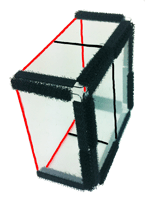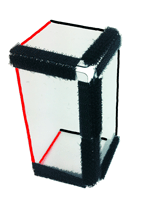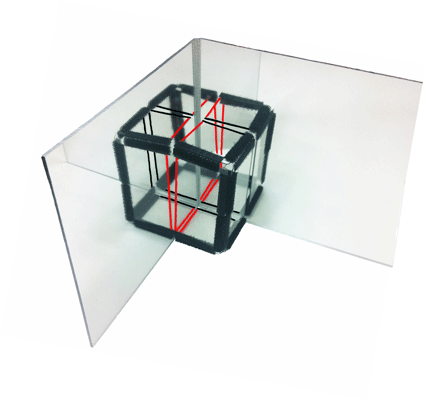The mirrors show two mutually perpendicular planes of mirror symmetry in cube Third mirror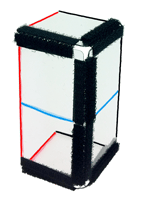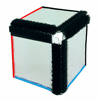The mirrors show three mutually perpendicular planes of mirror symmetry in cube Move of one mirror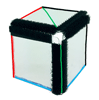The mirrors show three planes of mirror symmetry in cube. The planes of mirror symmetry are no longer all perpendicular. Two planes cross at angle 45deg and the third is perpendicular to both.